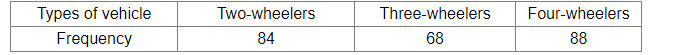# On a particular day, in a city, 240 vehicles of various types going past a crossing during a time interval were observed, as shown:Question:

On a particular day, in a city, 240 vehicles of various types going past a crossing during a time interval were observed, as shown:Out of these vehicles, one is chosen at random. What is the probability that the given vehicle is a two-wheeler?

Solution:

Total number of vehicles going past the crossing = 240
Number of two-wheelers = 84

Let E be the event that the selected vehicle is a two-wheeler. Then,

required probability $=P(E)=\frac{84}{240}=0.35$